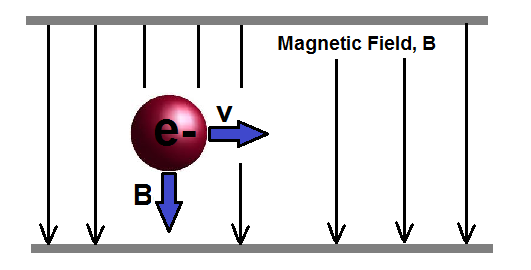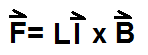﻿ Magnetic Force Calculator ﻿# Magnetic Force Calculator

### Magnetic Force Calculator of a Moving Charge Through a Magnetic FieldEnter Charge, q: C (coulombs) mC (millicoulombs) µC (microcoulombs) nC (nanocoulombs) pC (picocoulombs) Enter Velocity Vector, v: i j k Enter Magnetic Field Vector, B: i j k

0i+0j+0k

This magnetic force calculator calculates the magnetic force which a moving charge exerts travelling through a magnetic field. The parameters which determine the magnitude of this magnetic force are the magnitude of the charge, the magnitude of the velocity vector, and the magnitude of the magnetic field, according to the formula shown above.

To use this calculator, a user just enters the charge, C, the velocity vector, V, and the magnetic field, B, and then clicks the 'Submit' button. The resultant value will be automatically computed and displayed below.

The magnetic force is directly proportional to the magnitude of the charge of the object, the magnitude of the velocity, and the magnitude of the magnetic field through which it is travelling. The greater the charge, velocity, and/or the magnetic field, the greater the magnetic force which the object applies. Conversely, the smaller the charge, velocity, and/or magnetic field, the smaller the magnetic force.

Magnetic force is a measure of the amount of force which a charge exerts in the magnetic field through which it is travelling through.

If you look in the above illustration, the vector, v, is the velocity, the vector, B, is the magnetic field, and the electron is the charged object representing q.

The velocity and magnetic field are vectors because they aren't just magnitudes. Remember, vectors are values that have magnitude and direction. When an object is travelling with a velocity, v, it is travelling at a given speed (magnitude) at a given direction. The magnetic field is the same. It has a certain strength pointing to a certain angle. This is why these values are entered as vector values. The resultant value of the calculation, magnetic force, is also a vector value. Force has a magnitude and direction.

This calculator can be used for 2D vectors or 3D vectors. If a user is using this calculator for 2D vectors, which are vectors with only two dimensions, then s/he only fills in the i and j fields and leave the third field, k, blank. If using this calculator for a 3D vector, then the user enters in all fields.

The magnetic force is calculated according to the formula, F= qv x B. This means that the cross product of the product of the charge and the velocity vector with the magnetic field vector gives the resultant. If you want more in-depth detail and the formula for how cross products are calculated, please visit the Cross Product Calculator of Vectors.

### Magnetic Force Calculator of Current Flowing Through Straight WireEnter Length, L: m (meters) cm (centimeters) mm (millimeters) Enter Current Vector, I: i j k Enter Magnetic Field Vector, B: i j k

0i+0j+0k

This magnetic force calculator calculates the magnetic force which a current exerts travelling through a straight wire. The parameters which determine the magnitude of this magnetic force are the length of the wire, the magnitude of the current vector, and the magnitude of the magnetic field vector, according to the formula, F= IL x B. This formula is the same formula as the above calculator, except it has different parameters.

To use this calculator, a user just enters the length, L, the current vector, V, and the magnetic field, B, and then clicks the 'Submit' button. The resultant value will be automatically computed and displayed below.

The magnetic force is directly proportional to the length of the wire, the magnitude of the current, and the magnitude of the magnetic field through which it is travelling. The greater the length, current, and/or the magnetic field, the greater the magnetic force which the current applies. Conversely, the smaller the length, current, and/or magnetic field, the smaller the magnetic force.

The current and magnetic field are vectors because they aren't just magnitudes. Remember, vectors are values that have magnitude and direction. When current is travelling through a wire, it is travelling at a given speed (magnitude) at a given direction. The magnetic field is the same. It has a certain strength pointing in a certain direction. This is why these values are entered as vector values. The resultant value of the calculation, magnetic force, is also a vector value. Force has a magnitude and direction, because it is given at a certain strength, or magnitude, at a certain direction.

This calculator can be used for 2D vectors or 3D vectors. If being used for 2D vectors, which are vectors with only two dimensions, then only the i and j fields get filled in and the third field, k, is left blank. If being used for a 3D vector, then the user enters in all fields.

Related Resources

﻿